# Color Object color formation The color C of

• Slides: 32Color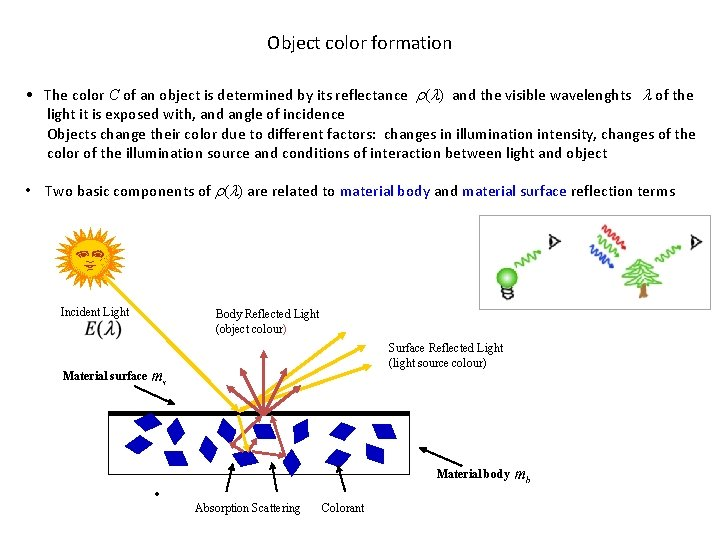Object color formation • The color C of an object is determined by its reflectance r(l) and the visible wavelenghts l of the light it is exposed with, and angle of incidence Objects change their color due to different factors: changes in illumination intensity, changes of the color of the illumination source and conditions of interaction between light and object • Two basic components of r(l) are related to material body and material surface reflection terms Incident Light Body Reflected Light (object colour) Surface Reflected Light (light source colour) Material surface Material body • Absorption Scattering Colorant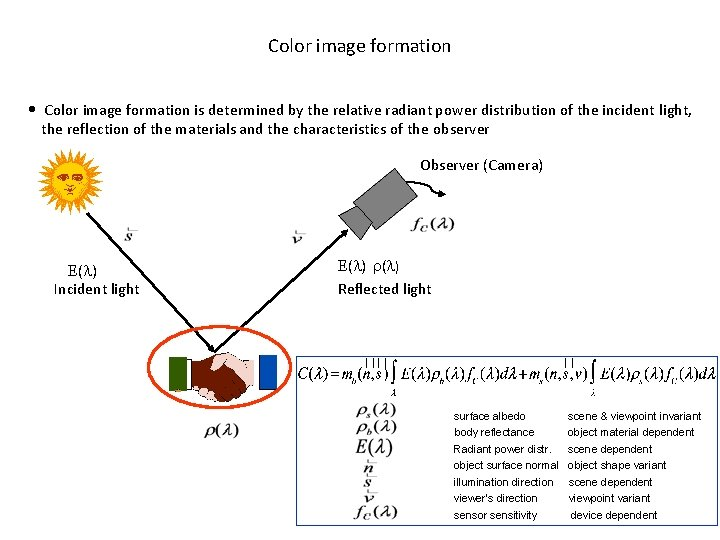Color image formation • Color image formation is determined by the relative radiant power distribution of the incident light, the reflection of the materials and the characteristics of the observer Observer (Camera) ( ) Incident light ( ) Reflected light surface albedo body reflectance Radiant power distr. object surface normal illumination direction viewer’s direction sensor sensitivity scene & viewpoint invariant object material dependent scene dependent object shape variant scene dependent viewpoint variant device dependentObserver/sensor sensitivity f ( ) • Reflected light spectrum i. e. every color can be represented by a 3 element vector, with values which are the proportions of each of the primary colors red (R), green (G) and blue (B) used to produce it. These are the tristimulus values Having the light spectrum and the spectral reflectance curve of the object, the appearance of the object depends on the spectral sensitivity of the observer Considering Tristimulus: RGB values of camera = Colour * Tristimulus. • Eye Response (cones only) Camera ResponseRadiant power distribution of the incident light ( ) • If the spectrum of the light source changes then the colour of the reflected light also changes.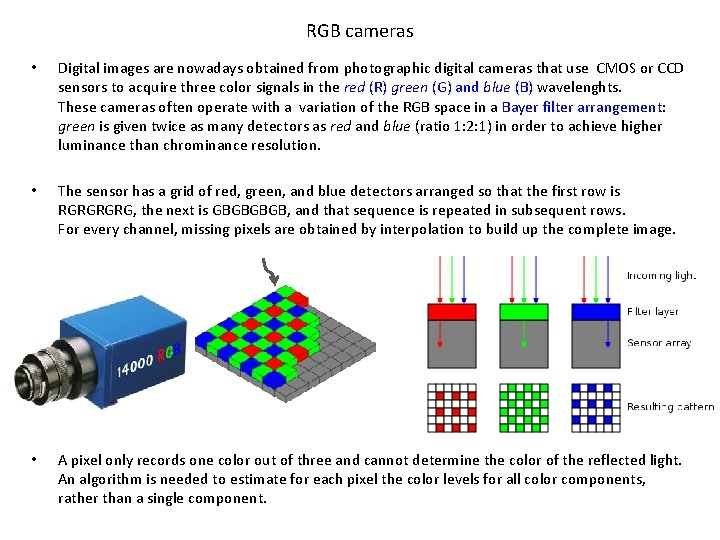RGB cameras • Digital images are nowadays obtained from photographic digital cameras that use CMOS or CCD sensors to acquire three color signals in the red (R) green (G) and blue (B) wavelenghts. These cameras often operate with a variation of the RGB space in a Bayer filter arrangement: green is given twice as many detectors as red and blue (ratio 1: 2: 1) in order to achieve higher luminance than chrominance resolution. • The sensor has a grid of red, green, and blue detectors arranged so that the first row is RGRG, the next is GBGB, and that sequence is repeated in subsequent rows. For every channel, missing pixels are obtained by interpolation to build up the complete image. • A pixel only records one color out of three and cannot determine the color of the reflected light. An algorithm is needed to estimate for each pixel the color levels for all color components, rather than a single component.Demosaicing algorithms original red green Bayer filter samples blue reconstructed after demosaicing • To obtain a full color image demosaicing algorithms are used that interpolate a set of complete green, red, blue values at each point. This is done in-camera producing a JPEG image. • Demosaicing can be done in different ways: – with bilinear interpolation the red value of a non-red pixel is computed as the average of the two or four adjacent red pixels, and similarly for blue and green – With the variable Number of Gradients interpolation, computing gradients near the pixel of interest and using the lower gradients representing smoother and more similar parts of the image to make an estimate – ….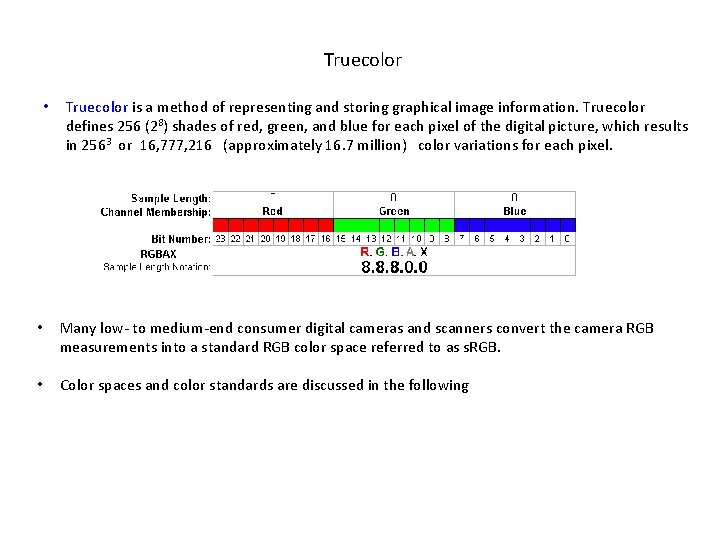Truecolor • Truecolor is a method of representing and storing graphical image information. Truecolor defines 256 (28) shades of red, green, and blue for each pixel of the digital picture, which results in 2563 or 16, 777, 216 (approximately 16. 7 million) color variations for each pixel. • Many low- to medium-end consumer digital cameras and scanners convert the camera RGB measurements into a standard RGB color space referred to as s. RGB. • Color spaces and color standards are discussed in the following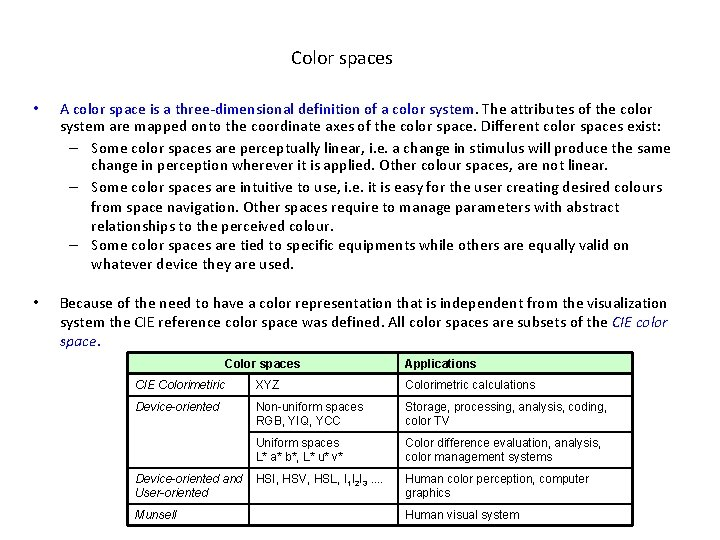Color spaces • A color space is a three-dimensional definition of a color system. The attributes of the color system are mapped onto the coordinate axes of the color space. Different color spaces exist: – Some color spaces are perceptually linear, i. e. a change in stimulus will produce the same change in perception wherever it is applied. Other colour spaces, are not linear. – Some color spaces are intuitive to use, i. e. it is easy for the user creating desired colours from space navigation. Other spaces require to manage parameters with abstract relationships to the perceived colour. – Some color spaces are tied to specific equipments while others are equally valid on whatever device they are used. • Because of the need to have a color representation that is independent from the visualization system the CIE reference color space was defined. All color spaces are subsets of the CIE color space. Color spaces Applications CIE Colorimetiric XYZ Colorimetric calculations Device-oriented Non-uniform spaces RGB, YIQ, YCC Storage, processing, analysis, coding, color TV Uniform spaces L* a* b*, L* u* v* Color difference evaluation, analysis, color management systems HSI, HSV, HSL, I 1 I 2 I 3. . Human color perception, computer graphics Device-oriented and User-oriented Munsell Human visual systemCIE color matching experiment • The first color matching experiments were devised in late 1920 s to characterize the relationship between the physical spectra and the perceived color, measuring the mixtures of different spectral distributions that are required for human observers to match colors • The experiments were conducted by using a circular split screen 2° in size (the angular size of the cone distribution in the human fovea). On one side of the field a test color was projected and on the other side, an observer-adjustable color was projected, that was a mixture of three monochromatic (single-wavelength) primary colors, each with fixed chromaticity, but with adjustable brightness • Not all test colors could be matched using this technique. When this was the case, a variable amount of one of the primaries was allowed to add to the test color. The amount of the primary added to the test color was considered to be a negative valueCIE RGB color space • In 1931 CIE standardized the RGB color matching functions obtained using three monochromatic primaries at wavelengths of 700 nm (red), 546. 1 nm (green) and 435. 8 nm (blue). The color matching functions are the amounts of primaries needed to match the monochromatic test primary at the wavelenght shown on the horizontal scale. Almost any spectral composition can be achieved by a suitably chosen linear mix of these three monochromatic primaries • Rather than specifying the brightness of each primary, the curves were normalized (scaled) to have constant area under them. The tabulated numerical values of these functions are known as the CIE standard observer (CIE 1931 2° standard observer). They roughly correspond to colour sensations of red, green and blue. • The CIE RGB color space is one of many RGB color spaces, distinguished by a particular set of monochromatic primary colors. The RGB tristimulus values for a color with a spectral power distribution I(λ) are given by integration of the scaled RGB color matching functions.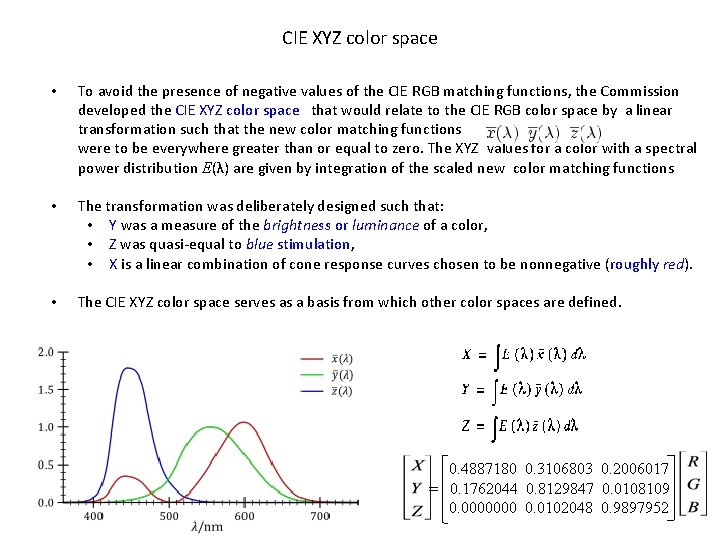CIE XYZ color space • To avoid the presence of negative values of the CIE RGB matching functions, the Commission developed the CIE XYZ color space that would relate to the CIE RGB color space by a linear transformation such that the new color matching functions were to be everywhere greater than or equal to zero. The XYZ values for a color with a spectral power distribution E(λ) are given by integration of the scaled new color matching functions • The transformation was deliberately designed such that: • Y was a measure of the brightness or luminance of a color, • Z was quasi-equal to blue stimulation, • X is a linear combination of cone response curves chosen to be nonnegative (roughly red). • The CIE XYZ color space serves as a basis from which other color spaces are defined. 0. 4887180 0. 3106803 0. 2006017 = 0. 1762044 0. 8129847 0. 0108109 0. 0000000 0. 0102048 0. 9897952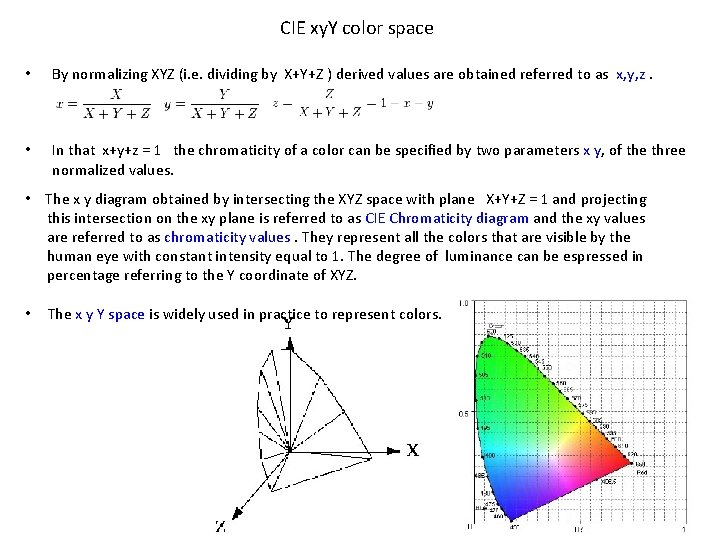CIE xy. Y color space • By normalizing XYZ (i. e. dividing by X+Y+Z ) derived values are obtained referred to as x, y, z. • In that x+y+z = 1 the chromaticity of a color can be specified by two parameters x y, of the three normalized values. • The x y diagram obtained by intersecting the XYZ space with plane X+Y+Z = 1 and projecting this intersection on the xy plane is referred to as CIE Chromaticity diagram and the xy values are referred to as chromaticity values. They represent all the colors that are visible by the human eye with constant intensity equal to 1. The degree of luminance can be espressed in percentage referring to the Y coordinate of XYZ. • The x y Y space is widely used in practice to represent colors.RGB color space • RGB coordinates can be directly derived from XYZ using a linear transformation. This is defined by fixing the coordinates of the white color and the three primaries. • The RGB space is usually represented by a cube using non-negative values within a 0– 1 range, assigning black to the origin at the vertex (0, 0, 0), and with increasing intensity values running along the three axes up to white at the vertex (1, 1, 1), diagonally opposite black. • An RGB triplet represents the point of the given color within the cube. Computations of the color similarity of two given RGB colors is obtained by simply calculating the distance between them.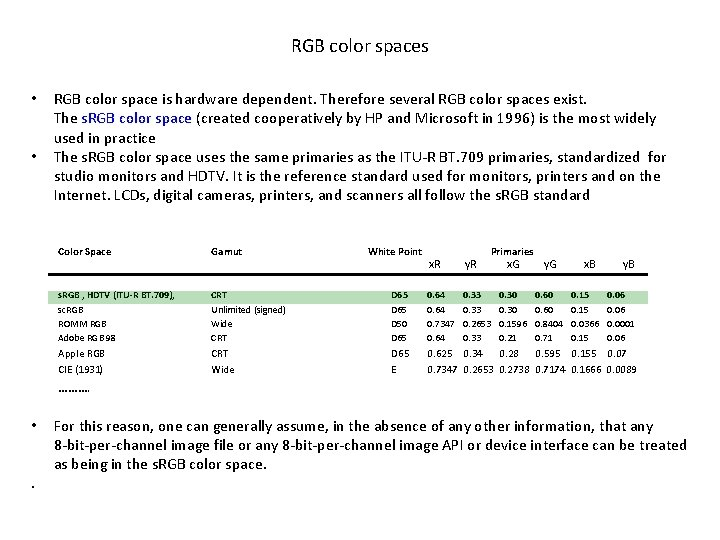RGB color spaces • • RGB color space is hardware dependent. Therefore several RGB color spaces exist. The s. RGB color space (created cooperatively by HP and Microsoft in 1996) is the most widely used in practice The s. RGB color space uses the same primaries as the ITU-R BT. 709 primaries, standardized for studio monitors and HDTV. It is the reference standard used for monitors, printers and on the Internet. LCDs, digital cameras, printers, and scanners all follow the s. RGB standard Color Space Gamut White Point Primaries x. R y. R x. G y. G x. B y. B • • • s. RGB , HDTV (ITU-R BT. 709), sc. RGB ROMM RGB Adobe RGB 98 CRT Unlimited (signed) Wide CRT D 65 D 50 D 65 0. 64 0. 7347 0. 64 • • Apple RGB CIE (1931) CRT Wide D 65 E 0. 625 0. 34 0. 28 0. 595 0. 155 0. 07 0. 7347 0. 2653 0. 2738 0. 7174 0. 1666 0. 0089 • ………. • 0. 33 0. 2653 0. 33 0. 30 0. 1596 0. 21 0. 60 0. 8404 0. 71 0. 15 0. 0366 0. 15 0. 06 0. 0001 0. 06 For this reason, one can generally assume, in the absence of any other information, that any 8 -bit-per-channel image file or any 8 -bit-per-channel image API or device interface can be treated as being in the s. RGB color space. .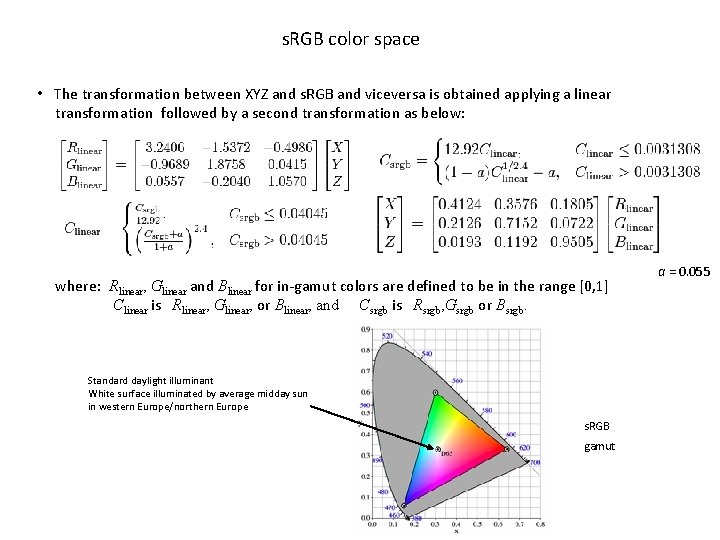s. RGB color space • The transformation between XYZ and s. RGB and viceversa is obtained applying a linear transformation followed by a second transformation as below: where: Rlinear, Glinear and Blinear for in-gamut colors are defined to be in the range [0, 1] Clinear is Rlinear, Glinear, or Blinear, and Csrgb is Rsrgb, Gsrgb or Bsrgb. Standard daylight illuminant White surface illuminated by average midday sun in western Europe/northern Europe s. RGB gamut a = 0. 055rgb color space • The rgb color space (RGB normalized) aims to separate the chromatic components from the brightness components. It is used to eliminate the influence of varying illumination. The red, green and blue channel can be transformed to their normalized counterpart r, g, b according to: • One of these normalised channels is redundant since r + g + b = 1. Therefore the normalised RGB space is sufficiently represented by two chromatic components (e. g. r, g) and a brightness componentOpponent color space • Human perception combines R, G and B response of the eye in opponent colors. Opponent colors can be hence expressed in RGB space. + Achromatic Red cone ++ + Rods + Red-Green + Green cone + Yellow Blue cone + Blue-Yellow l l l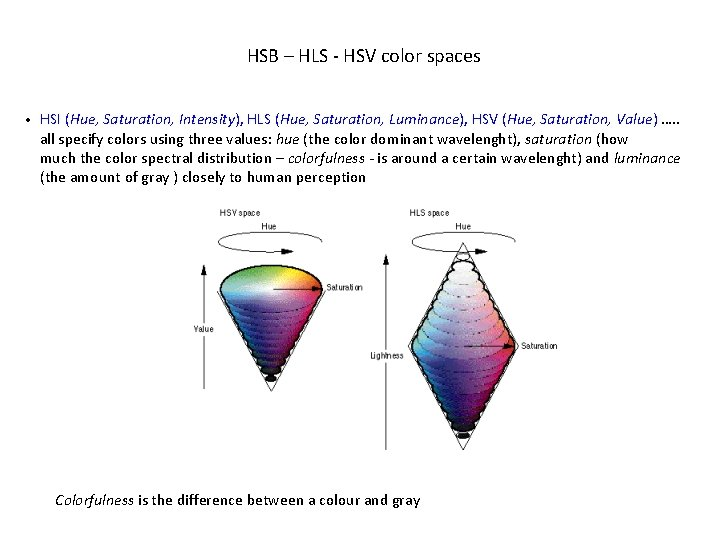HSB – HLS - HSV color spaces HSI (Hue, Saturation, Intensity), HLS (Hue, Saturation, Luminance), HSV (Hue, Saturation, Value) …. . all specify colors using three values: hue (the color dominant wavelenght), saturation (how much the color spectral distribution – colorfulness - is around a certain wavelenght) and luminance (the amount of gray ) closely to human perception • Colorfulness is the difference between a colour and grayHSV color space • HSV can be represented both as a cylinder or a cone space depending on whether the term S refers to: - saturation: colorfulness wrt its own brightness) - chroma: colorfulness wrt the brightness of another colour which appears white under similar viewing conditions • Values can be derived by the RGB values: Max = max (R, G, B); Min = min (R, G, B); V - Value = max (R, G, B); S - Chroma if (Max = 0) then Chroma= 0; else Chroma = (Max - Min) / Max; H - Hue if (Max = Min) then Hue is undefined (achromatic color); otherwise: if (Max = R & G > B) Hue = 60 * (G - B) / (Max-Min) else if (Max = R & G < B) Hue = 360 + 60 * (G - B) / (Max - Min) else if (G = Max) Hue = 60 * (2. 0 + (B - R) / (Max - Min)) else Hue = 60 * (4. 0 + (R - G) / (Max - Min)) Green (120 o) Cyan Yellow (60 o) (180 o) Red (0 o) Blue (240 o) Magenta (300 o) Value White Hue Black Chroma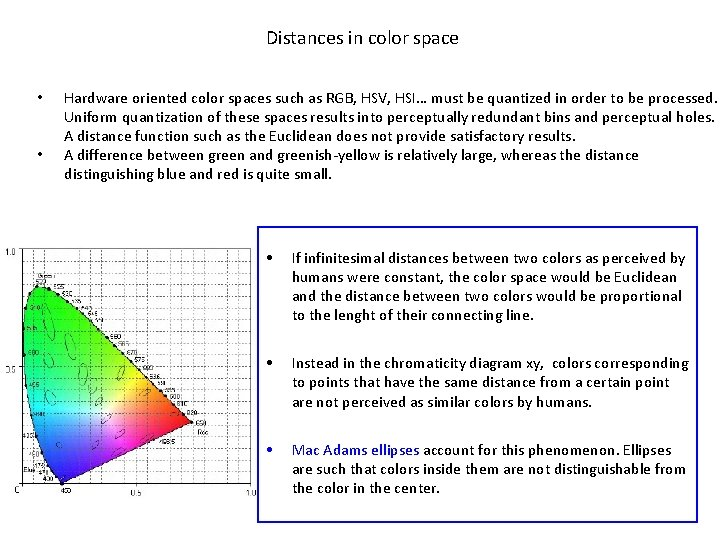Distances in color space • • Hardware oriented color spaces such as RGB, HSV, HSI… must be quantized in order to be processed. Uniform quantization of these spaces results into perceptually redundant bins and perceptual holes. A distance function such as the Euclidean does not provide satisfactory results. A difference between green and greenish-yellow is relatively large, whereas the distance distinguishing blue and red is quite small. • If infinitesimal distances between two colors as perceived by humans were constant, the color space would be Euclidean and the distance between two colors would be proportional to the lenght of their connecting line. • Instead in the chromaticity diagram xy, colors corresponding to points that have the same distance from a certain point are not perceived as similar colors by humans. • Mac Adams ellipses account for this phenomenon. Ellipses are such that colors inside them are not distinguishable from the color in the center.Perceptual color spaces • CIE solved this problem in 1976 with the development of the L*a*b* perceptual color space. Other perceptual spaces are L*u*v* and L*c*h*. All are based on transformations that approximate the XYZ Riemann space into an Euclidean space. These spaces are less distorted than CIE XYZ space although they are not completely free of distortion (Mac Adam’s ellipses become nearly circular here). • In the L*a*b* and L*u*v* color spaces distances can be computed as Euclidean distances: D(c 1, c 2) = [ (L*1 - L*2)2 + (a*1 - a*2)2 + (b*1 – b*2)2 ] 1/2 D(c 1, c 2) = [ (L*1 - L*2)2 + (u*1 - u*2)2 + (v*1 – v*2)2 ] 1/2 • Mathematical approximations introduced cause deviations from this property in certain parts of the spaces: – In L*u*v* Red is more represented than Green and Blue. – In L*a*b* there is a greater sensibility to Green than to Red and Blue is however more represented than in L*u*v*. L*a*b* L*u*v*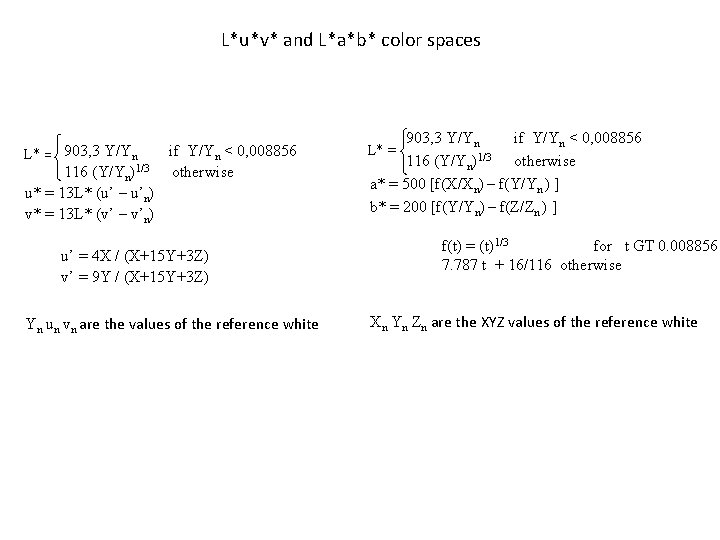L*u*v* and L*a*b* color spaces if Y/Yn < 0, 008856 L* = 903, 3 Y/Yn 116 (Y/Yn)1/3 otherwise u* = 13 L* (u’ – u’n) v* = 13 L* (v’ – v’n) u’ = 4 X / (X+15 Y+3 Z) v’ = 9 Y / (X+15 Y+3 Z) Yn un vn are the values of the reference white 903, 3 Y/Yn if Y/Yn < 0, 008856 116 (Y/Yn)1/3 otherwise a* = 500 [f(X/Xn) – f(Y/Yn ) ] b* = 200 [f(Y/Yn) – f(Z/Zn ) ] L* = f(t) = (t)1/3 for t GT 0. 008856 7. 787 t + 16/116 otherwise Xn Yn Zn are the XYZ values of the reference white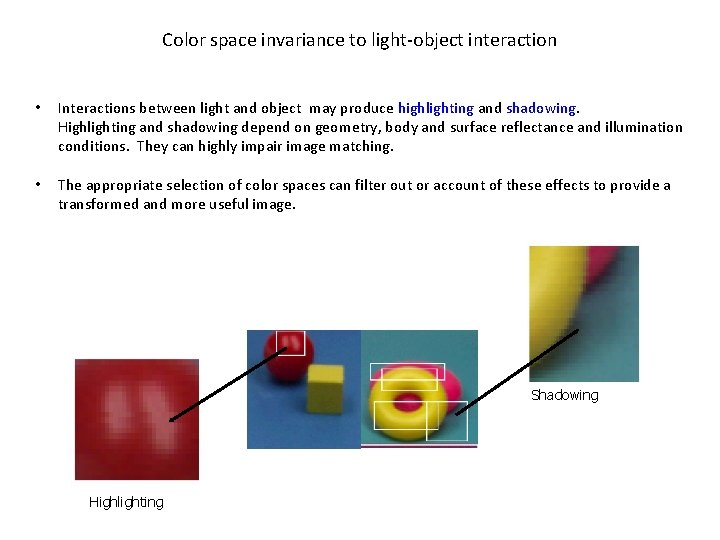Color space invariance to light-object interaction • Interactions between light and object may produce highlighting and shadowing. Highlighting and shadowing depend on geometry, body and surface reflectance and illumination conditions. They can highly impair image matching. • The appropriate selection of color spaces can filter out or account of these effects to provide a transformed and more useful image. Shadowing Highlighting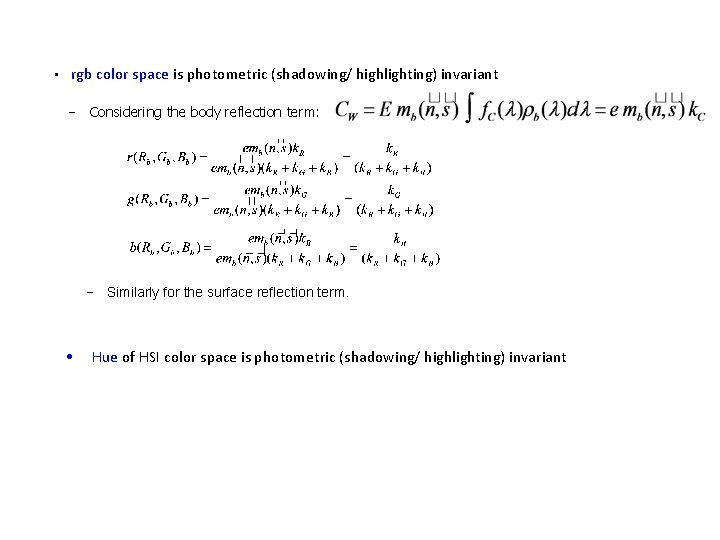• rgb color space is photometric (shadowing/ highlighting) invariant − Considering the body reflection term: − Similarly for the surface reflection term. • Hue of HSI color space is photometric (shadowing/ highlighting) invariantl 1 l 2 l 3 color space is obtained from RGB manipulation and is invariant to highlighting effects of light interaction particularly for shiny objects • Original RGB image 3 D plot of RGB image l 1 l 2 l 3 image 3 D plot of l 1 l 2 l 3 imagec 1 c 2 c 3 color space • c 1 c 2 c 3 color space is obtained from RGB manipulation and is invariant to shadowing effects of light interaction particularly for matte objects 3 D plot of RGB image 3 D plot of c 1 c 2 c 3 image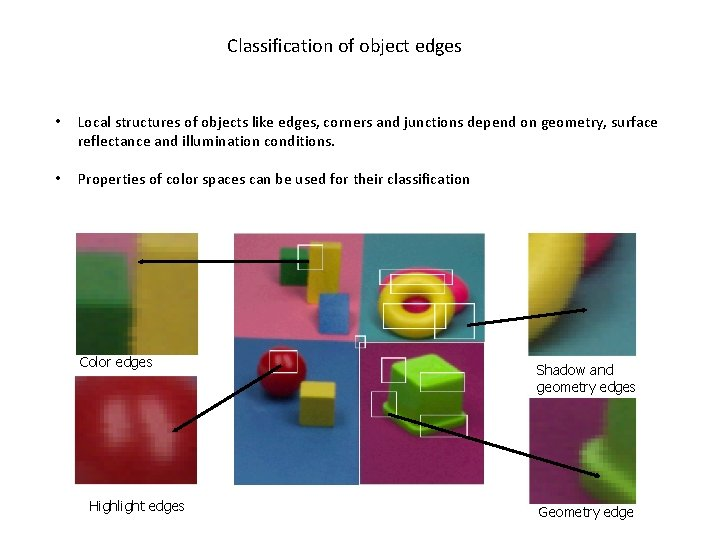Classification of object edges • Local structures of objects like edges, corners and junctions depend on geometry, surface reflectance and illumination conditions. • Properties of color spaces can be used for their classification Color edges Highlight edges Shadow and geometry edges Geometry edgeColor edge maxima by type shadows and geometry highlights colour edges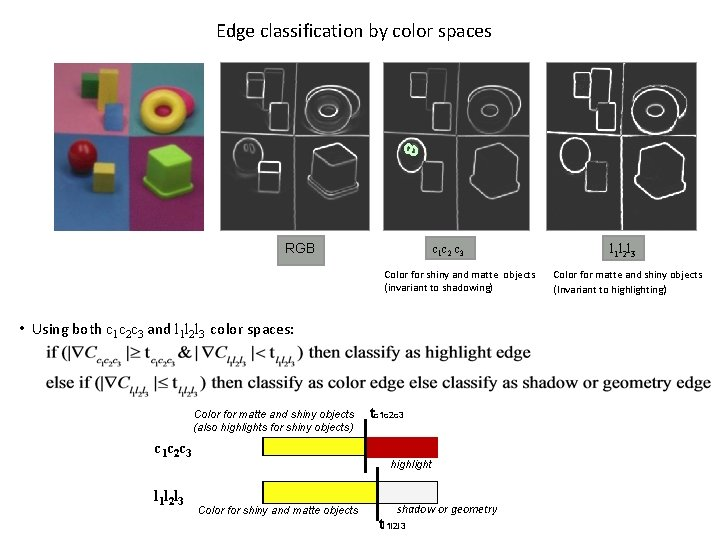Edge classification by color spaces RGB c 1 c 2 c 3 Color for shiny and matte objects (invariant to shadowing) • Using both c 1 c 2 c 3 and l 1 l 2 l 3 color spaces: Color for matte and shiny objects (also highlights for shiny objects) c 1 c 2 c 3 l 1 l 2 l 3 tc 1 c 2 c 3 highlight Color for shiny and matte objects shadow or geometry tl 1 l 2 l 3 Color for matte and shiny objects (Invariant to highlighting)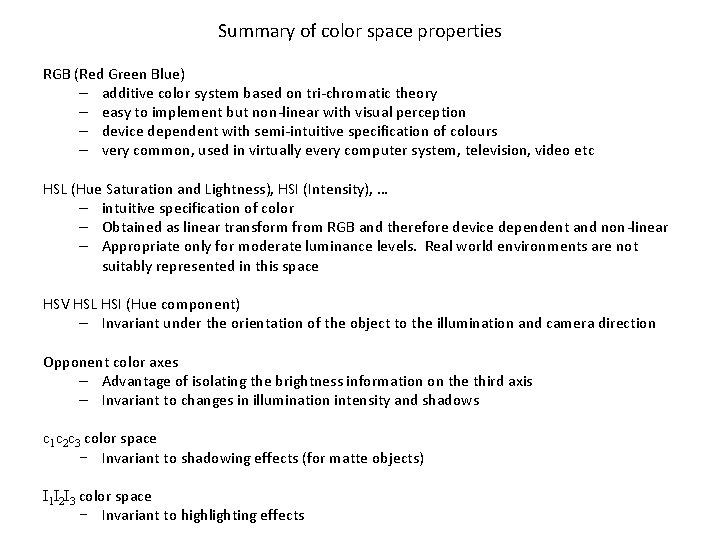Summary of color space properties RGB (Red Green Blue) – additive color system based on tri-chromatic theory – easy to implement but non-linear with visual perception – device dependent with semi-intuitive specification of colours – very common, used in virtually every computer system, television, video etc HSL (Hue Saturation and Lightness), HSI (Intensity), … – intuitive specification of color – Obtained as linear transform from RGB and therefore device dependent and non-linear – Appropriate only for moderate luminance levels. Real world environments are not suitably represented in this space HSV HSL HSI (Hue component) – Invariant under the orientation of the object to the illumination and camera direction Opponent color axes – Advantage of isolating the brightness information on the third axis – Invariant to changes in illumination intensity and shadows c 1 c 2 c 3 color space − Invariant to shadowing effects (for matte objects) I 1 I 2 I 3 color space − Invariant to highlighting effectsCIE L*u*v* – based directly on CIE XYZ, attempts to linearize the perceptibility of unit vector color differences. Non-linear transformation but the conversions are reversible – The non-linear relationship for L*u*v* is intended to mimic the logarithmic response of the eye • CIE L*a*b* – based directly on CIE XYZ, attempts to linearise the perceptibility of unit vector color differences. Non-linear transformation but the conversions are reversible – The non-linear relationships for L*a* and b* are intended to mimic the logarithmic response of the eye – Suitable for real world scene color representation